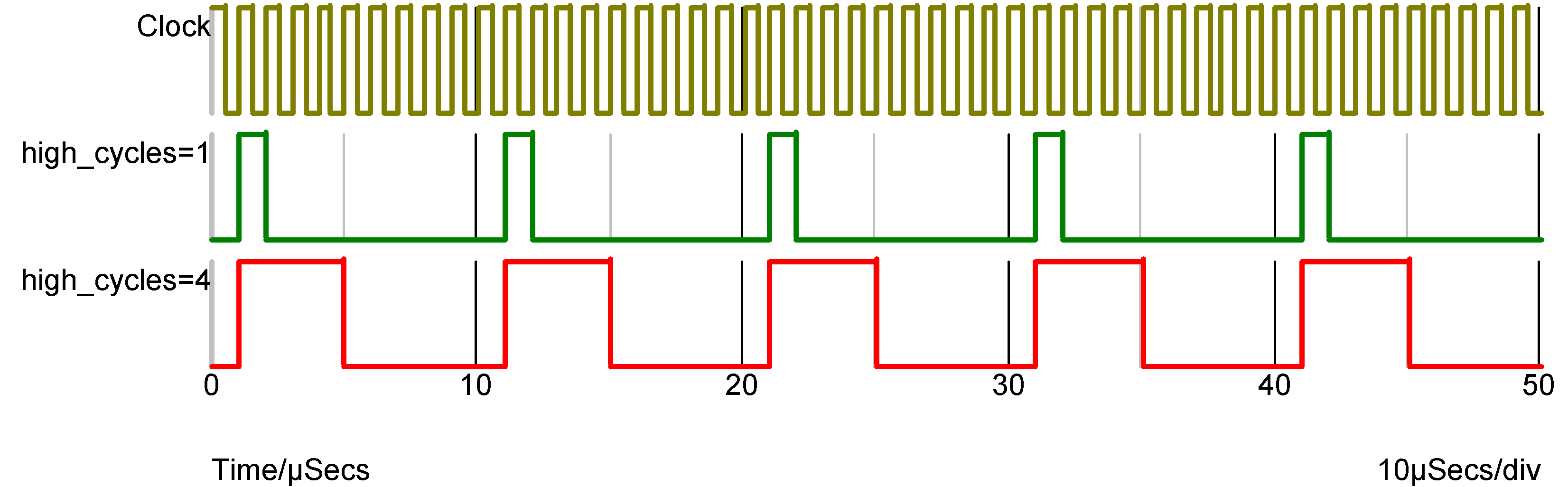# Frequency DividerIn this topic:

## Netlist entry

Axxxx freq_in freq_out model_name

## Connection details

 Name Description Flow Type freq_in Frequency input in d freq_out Frequency output out d

## Model format

.MODEL model_name d_fdiv parameters

## Model parameters

 Name Description Type Default Limits div_factor Divide factor integer 2 $1 - \infty$ high_cycles Number of high clock cycles integer 1 $1 - \infty$ i_count Output initial count value integer 0 $0 - \infty$ rise_delay Rise delay real 1nS 1e-12 $- \infty$ fall_delay Fall delay real 1nS 1e-12 $- \infty$ freq_in_load Freq_in load value (F) real 1pF none family Logic family string UNIV none in_family Input logic family string UNIV none out_family Output logic family string UNIV none out_res Digital output resistance real 100 $0 - \infty$ out_res_pos Digital output res. pos. slope real out_res $0 - \infty$ out_res_neg Digital output res. neg. slope out_res $0 - \infty$ min_sink Minimum sink current real -0.001 none max_source Maximum source current real 0.001 none sink_current Input sink current real 0 none source_current Input source current real 0 none

## Device Operation

This device is a positive edge triggered frequency divider. Three model parameters allow arbitrary definition of the divide ratio, output duty cycle, output phase and initial delay. Operation of the frequency divider is illustrated by the following diagram which shows the output of a frequency divider with a DIV_FACTOR of 10 and two alternative values of HIGH_CYCLES.The above was carried out with I_COUNT=0. I_COUNT is the initial value of the internal counter. The output first goes high when it attains a value of 1 or 1+DIVIDE_RATIO so when I_COUNT is zero (the default) the output first goes high after the first rising edge. If I_COUNT is set to 5 the output first goes high after the 6th rising edge and if I_COUNT is -20, the 21st rising edge.